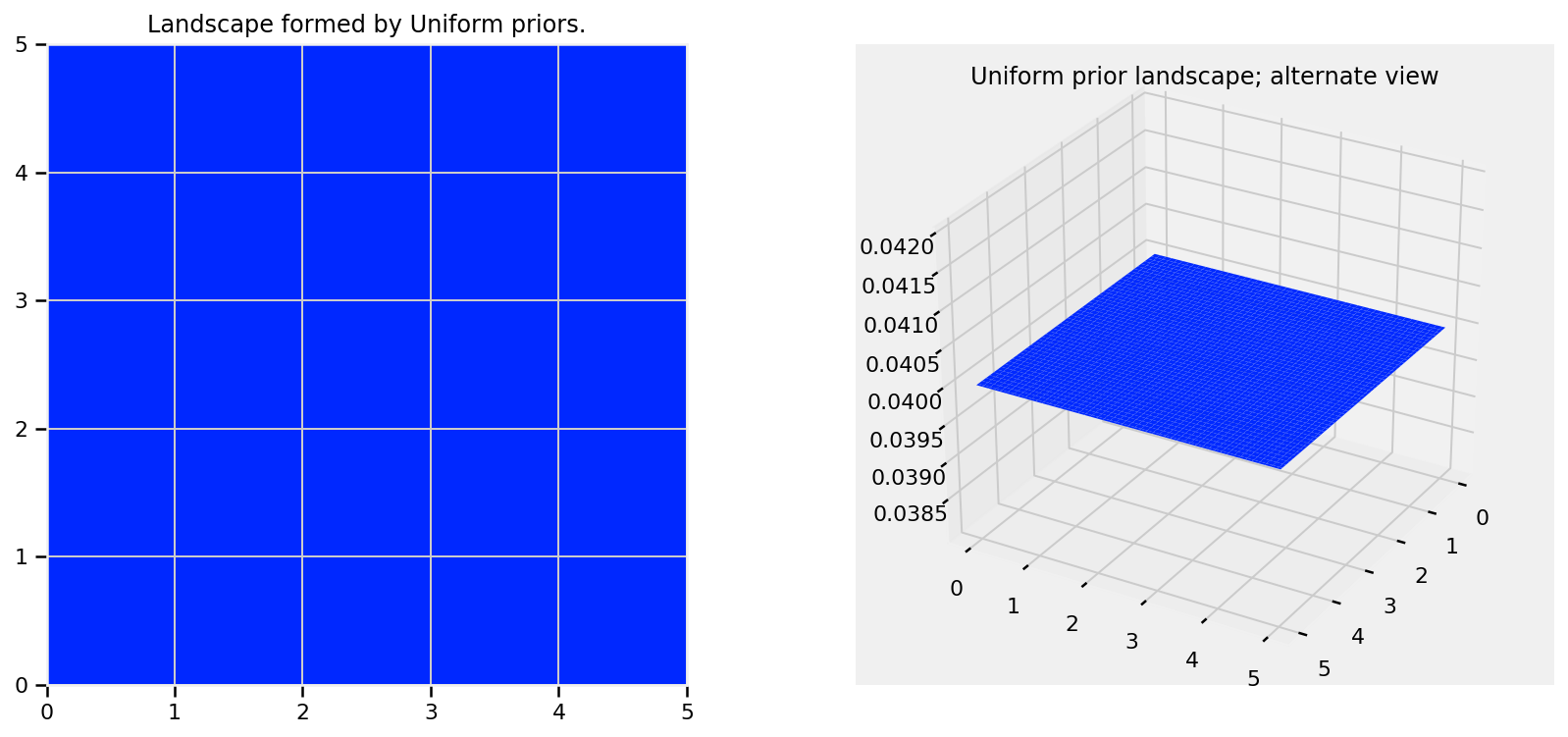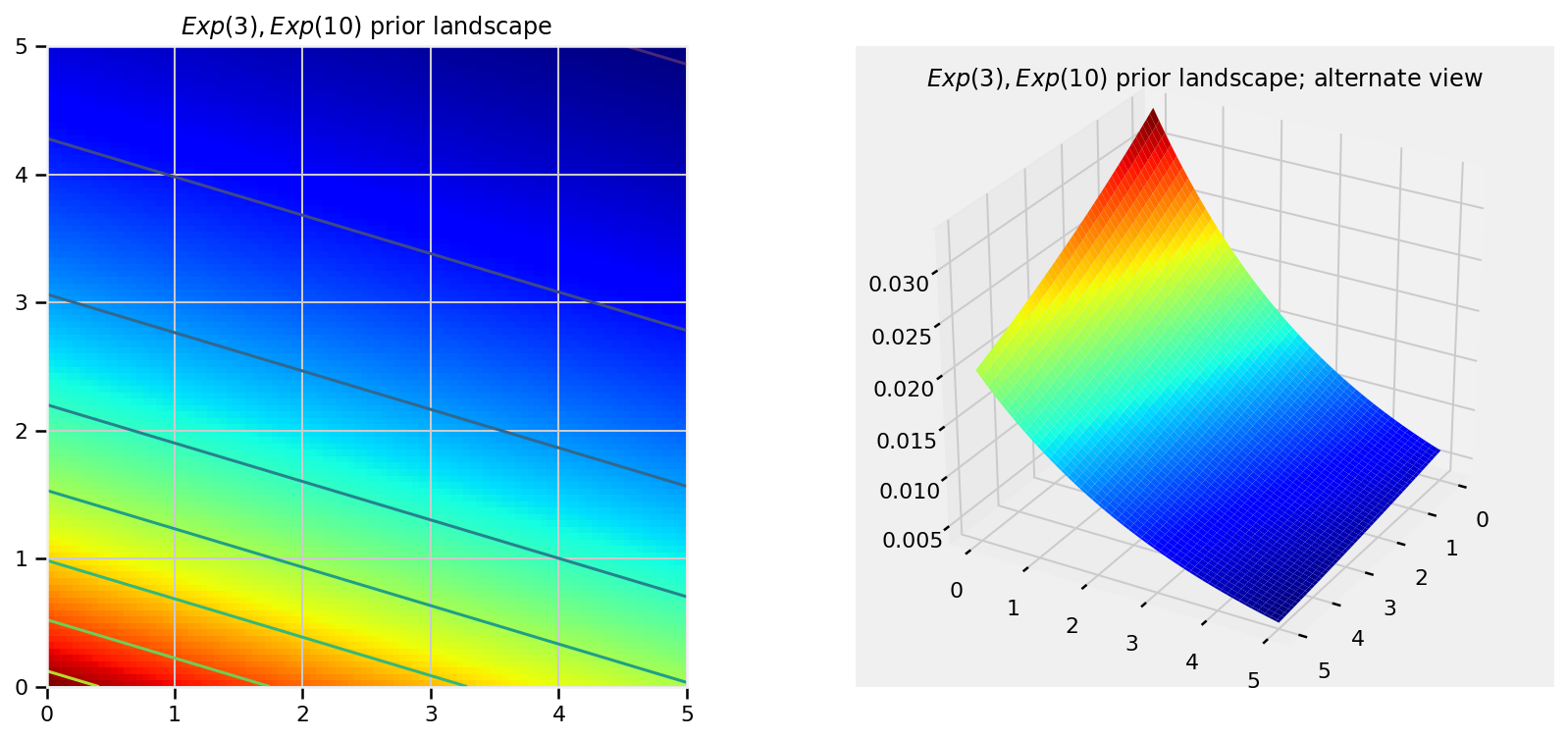# Probabilistic Programming and Bayesian Methods for Hackers Chapter 3¶

Original content (this Jupyter notebook) created by Cam Davidson-Pilon (@Cmrn_DP)

Ported to Tensorflow Probability by Matthew McAteer (@MatthewMcAteer0), with help from Bryan Seybold, Mike Shwe (@mikeshwe), Josh Dillon, and the rest of the TFP team at Google ([email protected]).

Welcome to Bayesian Methods for Hackers. The full Github repository is available at github/Probabilistic-Programming-and-Bayesian-Methods-for-Hackers. The other chapters can be found on the project's homepage. We hope you enjoy the book, and we encourage any contributions!

### Table of Contents¶

• Dependencies & Prerequisites
• Opening the black box of MCMC
• The Bayesian landscape
• Exploring the landscape using the MCMC
• Why Thousands of Samples?
• Algorithms to perform MCMC
• Other aproximation solutions to the posterior
• Example: Unsupervised Clustering Using a Mixture Model
• Cluster Investigation
• Important: Don't mix posterior samples
• Returning to Clustering: Prediction
• Diagnosing Convergence
• Autocorrelation
• How does this relate to MCMC convergence?
• Thinning
• Additional Plotting Options
• Useful tips for MCMC
• Intelligent starting values
• Priors
• Covariance matrices and eliminating parameters
• The Folk Theorem of Statistical Computing
• Conclusion
• References

### Dependencies & Prerequisites¶

Tensorflow Probability is part of the colab default runtime, so you don't need to install Tensorflow or Tensorflow Probability if you're running this in the colab.
If you're running this notebook in Jupyter on your own machine (and you have already installed Tensorflow), you can use the following
• For the most recent nightly installation: pip3 install -q tfp-nightly
• For the most recent stable TFP release: pip3 install -q --upgrade tensorflow-probability
• For the most recent stable GPU-connected version of TFP: pip3 install -q --upgrade tensorflow-probability-gpu
• For the most recent nightly GPU-connected version of TFP: pip3 install -q tfp-nightly-gpu
In summary, if you are running this in a Colab, Tensorflow and TFP are already installed
In :
#@title Imports and Global Variables  { display-mode: "form" }
"""
The book uses a custom matplotlibrc file, which provides the unique styles for
matplotlib plots. If executing this book, and you wish to use the book's
styling, provided are two options:
1. Overwrite your own matplotlibrc file with the rc-file provided in the
book's styles/ dir. See http://matplotlib.org/users/customizing.html
2. Also in the styles is  bmh_matplotlibrc.json file. This can be used to
update the styles in only this notebook. Try running the following code:

import json
s = json.load(open("../styles/bmh_matplotlibrc.json"))
matplotlib.rcParams.update(s)
"""
!pip3 install -q pandas_datareader
!pip3 install -q wget
from __future__ import absolute_import, division, print_function

#@markdown This sets the warning status (default is ignore, since this notebook runs correctly)
warning_status = "ignore" #@param ["ignore", "always", "module", "once", "default", "error"]
import warnings
warnings.filterwarnings(warning_status)
with warnings.catch_warnings():
warnings.filterwarnings(warning_status, category=DeprecationWarning)
warnings.filterwarnings(warning_status, category=UserWarning)

import numpy as np
import os
#@markdown This sets the styles of the plotting (default is styled like plots from [FiveThirtyeight.com](https://fivethirtyeight.com/))
matplotlib_style = 'fivethirtyeight' #@param ['fivethirtyeight', 'bmh', 'ggplot', 'seaborn', 'default', 'Solarize_Light2', 'classic', 'dark_background', 'seaborn-colorblind', 'seaborn-notebook']
import matplotlib as mpl
import matplotlib.pyplot as plt; plt.style.use(matplotlib_style)
import matplotlib.axes as axes;
from matplotlib.patches import Ellipse
from mpl_toolkits.mplot3d import Axes3D
import pandas_datareader.data as web
%matplotlib inline
import seaborn as sns; sns.set_context('notebook')
from IPython.core.pylabtools import figsize
#@markdown This sets the resolution of the plot outputs (retina is the highest resolution)
notebook_screen_res = 'retina' #@param ['retina', 'png', 'jpeg', 'svg', 'pdf']
%config InlineBackend.figure_format = notebook_screen_res

import tensorflow as tf
tfe = tf.contrib.eager

# Eager Execution
#@markdown Check the box below if you want to use [Eager Execution](https://www.tensorflow.org/guide/eager)
#@markdown Eager execution provides An intuitive interface, Easier debugging, and a control flow comparable to Numpy. You can read more about it on the [Google AI Blog](https://ai.googleblog.com/2017/10/eager-execution-imperative-define-by.html)
use_tf_eager = False #@param {type:"boolean"}

# Use try/except so we can easily re-execute the whole notebook.
if use_tf_eager:
try:
tf.enable_eager_execution()
except:
pass

import tensorflow_probability as tfp
tfd = tfp.distributions
tfb = tfp.bijectors

def evaluate(tensors):
"""Evaluates Tensor or EagerTensor to Numpy ndarrays.
Args:
tensors: Object of Tensor or EagerTensors; can be list, tuple,
namedtuple or combinations thereof.

Returns:
ndarrays: Object with same structure as tensors except with Tensor or
EagerTensors replaced by Numpy ndarrays.
"""
if tf.executing_eagerly():
return tf.contrib.framework.nest.pack_sequence_as(
tensors,
[t.numpy() if tf.contrib.framework.is_tensor(t) else t
for t in tf.contrib.framework.nest.flatten(tensors)])
return sess.run(tensors)

class _TFColor(object):
"""Enum of colors used in TF docs."""
red = '#F15854'
blue = '#5DA5DA'
orange = '#FAA43A'
green = '#60BD68'
pink = '#F17CB0'
brown = '#B2912F'
purple = '#B276B2'
yellow = '#DECF3F'
gray = '#4D4D4D'
def __getitem__(self, i):
return [
self.red,
self.orange,
self.green,
self.blue,
self.pink,
self.brown,
self.purple,
self.yellow,
self.gray,
][i % 9]
TFColor = _TFColor()

def session_options(enable_gpu_ram_resizing=True, enable_xla=True):
"""
Allowing the notebook to make use of GPUs if they're available.

XLA (Accelerated Linear Algebra) is a domain-specific compiler for linear
algebra that optimizes TensorFlow computations.
"""
config = tf.ConfigProto()
config.log_device_placement = True
if enable_gpu_ram_resizing:
# allow_growth=True makes it possible to connect multiple colabs to your
# GPU. Otherwise the colab malloc's all GPU ram.
config.gpu_options.allow_growth = True
if enable_xla:
# Enable on XLA. https://www.tensorflow.org/performance/xla/.
config.graph_options.optimizer_options.global_jit_level = (
tf.OptimizerOptions.ON_1)
return config

def reset_sess(config=None):
"""
Convenience function to create the TF graph & session or reset them.
"""
if config is None:
config = session_options()
global sess
tf.reset_default_graph()
try:
sess.close()
except:
pass
sess = tf.InteractiveSession(config=config)

reset_sess()


## Opening the black box of MCMC¶

The previous two chapters hid the inner-mechanics of TFP, and more generally Markov Chain Monte Carlo (MCMC), from the reader. The reason for including this chapter is three-fold. The first is that any book on Bayesian inference must discuss MCMC. I cannot fight this. Blame the statisticians. Secondly, knowing the process of MCMC gives you insight into whether your algorithm has converged(Converged to what? We will get to that). Thirdly, we'll understand why we are returned thousands of samples from the posterior as a solution, which at first thought can be odd.

### The Bayesian landscape¶

When we setup a Bayesian inference problem with $N$ unknowns, we are implicitly creating an $N$ dimensional space for the prior distributions to exist in. Associated with the space is an additional dimension, which we can describe as the surface, or curve, that sits on top of the space, that reflects the prior probability of a particular point. The surface on the space is defined by our prior distributions. For example, if we have two unknowns $p_1$ and $p_2$, and priors for both are $\text{Uniform}(0,5)$, the space created is a square of length 5 and the surface is a flat plane that sits on top of the square (representing that every point is equally likely).

In :
x_ = y_ = np.linspace(0., 5., 100., dtype=np.float32)
X_, Y_ = evaluate(tf.meshgrid(x_, y_))

uni_x_ = evaluate(tfd.Uniform(low=0., high=5.).prob(x_))
m_ = np.median(uni_x_)

uni_y_ = evaluate(tfd.Uniform(low=0., high=5.).prob(y_))
M_ = evaluate(tf.matmul(tf.expand_dims(uni_x_, 1), tf.expand_dims(uni_y_, 0)))

plt.figure(figsize(12.5, 6))
jet = plt.cm.jet
fig = plt.figure()
plt.subplot(121)

im = plt.imshow(M_, interpolation='none', origin='lower',
cmap=jet, vmax=1, vmin=-.15, extent=(0, 5, 0, 5))

plt.xlim(0, 5)
plt.ylim(0, 5)
plt.title("Landscape formed by Uniform priors.")

ax = fig.add_subplot(122, projection='3d')
ax.plot_surface(X_, Y_, M_, cmap=plt.cm.jet, vmax=1, vmin=-.15)
ax.view_init(azim=390)
plt.title("Uniform prior landscape; alternate view");

<Figure size 900x432 with 0 Axes>Alternatively, if the two priors are $\text{Exp}(3)$ and $\text{Exp}(10)$, then the space is all positive numbers on the 2-D plane, and the surface induced by the priors looks like a water fall that starts at the point (0,0) and flows over the positive numbers.

The plots below visualize this. The more dark red the color, the more prior probability is assigned to that location. Conversely, areas with darker blue represent that our priors assign very low probability to that location.

In :
exp_x_ = evaluate(tfd.Exponential(rate=(1./3.)).prob(x_))
exp_y_ = evaluate(tfd.Exponential(rate=(1./10.)).prob(y_))

M_ = evaluate(tf.matmul(tf.expand_dims(exp_x_, 1), tf.expand_dims(exp_y_, 0)))

plt.figure(figsize(12.5, 6))
jet = plt.cm.jet
fig = plt.figure()
plt.subplot(121)
CS = plt.contour(X_, Y_, M_)
im = plt.imshow(M_, interpolation='none', origin='lower',
cmap=jet, extent=(0, 5, 0, 5))
plt.xlim(0, 5)
plt.ylim(0, 5)
plt.title(r"$Exp(3), Exp(10)$ prior landscape")

ax = fig.add_subplot(122, projection='3d')
ax.plot_surface(X_, Y_, M_, cmap=plt.cm.jet)
ax.view_init(azim=30)
plt.title(r"$Exp(3), Exp(10)$ prior landscape; alternate view");

<Figure size 900x432 with 0 Axes>These are simple examples in 2D space, where our brains can understand surfaces well. In practice, spaces and surfaces generated by our priors can be much higher dimensional.

If these surfaces describe our prior distributions on the unknowns, what happens to our space after we incorporate our observed data $X$? The data $X$ does not change the space, but it changes the surface of the space by pulling and stretching the fabric of the prior surface to reflect where the true parameters likely live. More data means more pulling and stretching, and our original shape becomes mangled or insignificant compared to the newly formed shape. Less data, and our original shape is more present. Regardless, the resulting surface describes the posterior distribution.

Again I must stress that it is, unfortunately, impossible to visualize this in large dimensions. For two dimensions, the data essentially pushes up the original surface to make tall mountains. The tendency of the observed data to push up the posterior probability in certain areas is checked by the prior probability distribution, so that less prior probability means more resistance. Thus in the double-exponential prior case above, a mountain (or multiple mountains) that might erupt near the (0,0) corner would be much higher than mountains that erupt closer to (5,5), since there is more resistance (low prior probability) near (5,5). The peak reflects the posterior probability of where the true parameters are likely to be found. Importantly, if the prior has assigned a probability of 0, then no posterior probability will be assigned there.

Suppose the priors mentioned above represent different parameters $\lambda$ of two Poisson distributions. We observe a few data points and visualize the new landscape:

In :
# creating the observed data
# sample size of data we observe, trying varying this (keep it less than 100 ;)
N = 1 #param {type:"slider", min:1, max:15, step:1}

# the true parameters, but of course we do not see these values...
lambda_1_true = float(1.)
lambda_2_true = float(3.)

#...we see the data generated, dependent on the above two values.
data = tf.concat([
tfd.Poisson(rate=lambda_1_true).sample(sample_shape=(N, 1), seed=4),
tfd.Poisson(rate=lambda_2_true).sample(sample_shape=(N, 1), seed=8)
], axis=1)
data_ = evaluate(data)
print("observed (2-dimensional,sample size = %d): \n" % N, data_)

# plotting details.
x_ = y_ = np.linspace(.01, 5, 100)

likelihood_x = tf.math.reduce_prod(tfd.Poisson(rate=x_).prob(data_[:,0][:,tf.newaxis]),axis=0)
likelihood_y = tf.math.reduce_prod(tfd.Poisson(rate=y_).prob(data_[:,1][:,tf.newaxis]),axis=0)

L_ = evaluate(tf.matmul(likelihood_x[:,tf.newaxis],likelihood_y[tf.newaxis,:]))

observed (2-dimensional,sample size = 1):
[[3. 4.]]

In :
plt.figure(figsize(12.5, 15.0))
# matplotlib heavy lifting below, beware!

# SUBPLOT for regular Uniform
plt.subplot(221)

uni_x_ = evaluate(tfd.Uniform(low=0., high=5.).prob(tf.cast(x_,dtype=tf.float32)))
m = np.median(uni_x_[uni_x_ > 0])
uni_x_[uni_x_ == 0] = m
uni_y_ = evaluate(tfd.Uniform(low=0., high=5.).prob(tf.cast(y_,dtype=tf.float32)))
m = np.median(uni_y_[uni_y_ > 0])
uni_y_[uni_y_ == 0] = m

M_ = evaluate(tf.matmul(tf.expand_dims(uni_x_, 1), tf.expand_dims(uni_y_, 0)))

im = plt.imshow(M_, interpolation='none', origin='lower',
cmap=jet, vmax=1, vmin=-.15, extent=(0, 5, 0, 5))
plt.scatter(lambda_2_true, lambda_1_true, c="k", s=50, edgecolor="none")
plt.xlim(0, 5)
plt.ylim(0, 5)
plt.title(r"Landscape formed by Uniform priors on $p_1, p_2$.")

# SUBPLOT for Uniform + Data point
plt.subplot(223)
plt.contour(x_, y_, M_ * L_)
im = plt.imshow(M_ * L_, interpolation='none', origin='lower',
cmap=jet, extent=(0, 5, 0, 5))
plt.title("Landscape warped by %d data observation;\n Uniform priors on $p_1, p_2$." % N)
plt.scatter(lambda_2_true, lambda_1_true, c="k", s=50, edgecolor="none")
plt.xlim(0, 5)
plt.ylim(0, 5)

# SUBPLOT for regular Exponential
plt.subplot(222)
exp_x_ = evaluate(tfd.Exponential(rate=.3).prob(tf.to_float(x_)))
exp_x_[np.isnan(exp_x_)] = exp_x_
exp_y_ = evaluate(tfd.Exponential(rate=.10).prob(tf.to_float(y_)))
exp_y_[np.isnan(exp_y_)] = exp_y_
M_ = evaluate(tf.matmul(tf.expand_dims(exp_x_, 1), tf.expand_dims(exp_y_, 0)))
plt.contour(x_, y_, M_)
im = plt.imshow(M_, interpolation='none', origin='lower',
cmap=jet, extent=(0, 5, 0, 5))
plt.scatter(lambda_2_true, lambda_1_true, c="k", s=50, edgecolor="none")
plt.xlim(0, 5)
plt.ylim(0, 5)
plt.title("Landscape formed by Exponential priors on $p_1, p_2$.")

# SUBPLOT for Exponential + Data point
plt.subplot(224)
# This is the likelihood times prior, that results in the posterior.
plt.contour(x_, y_, M_ * L_)
im = plt.imshow(M_ * L_, interpolation='none', origin='lower',
cmap=jet, extent=(0, 5, 0, 5))

plt.scatter(lambda_2_true, lambda_1_true, c="k", s=50, edgecolor="none")
plt.title("Landscape warped by %d data observation;\n Exponential priors on \
$p_1, p_2$." % N)
plt.xlim(0, 5)
plt.ylim(0, 5);
`